# Selina Solutions Concise Maths Class 10 Chapter 18 Tangents and Intersecting Chords Exercise 18(B)

Theorems on intersecting chords (internally and externally) and the angle between a tangent and chord through the point of contact are the key concepts discussed in this exercise. Students searching for a reference to solving problems can end their search as the Selina Solutions for Class 10 Maths is the one resource for this purpose. Further, the solutions are as per the latest ICSE marking schemes. The Concise Selina Solutions for Class 10 Maths Chapter 18 Tangents and Intersecting Chords Exercise 18(B) solution PDF can be downloaded effortlessly from the link below.

## Selina Solutions Concise Maths Class 10 Chapter 18 Tangents and Intersecting Chords Exercise 18(B) Download PDF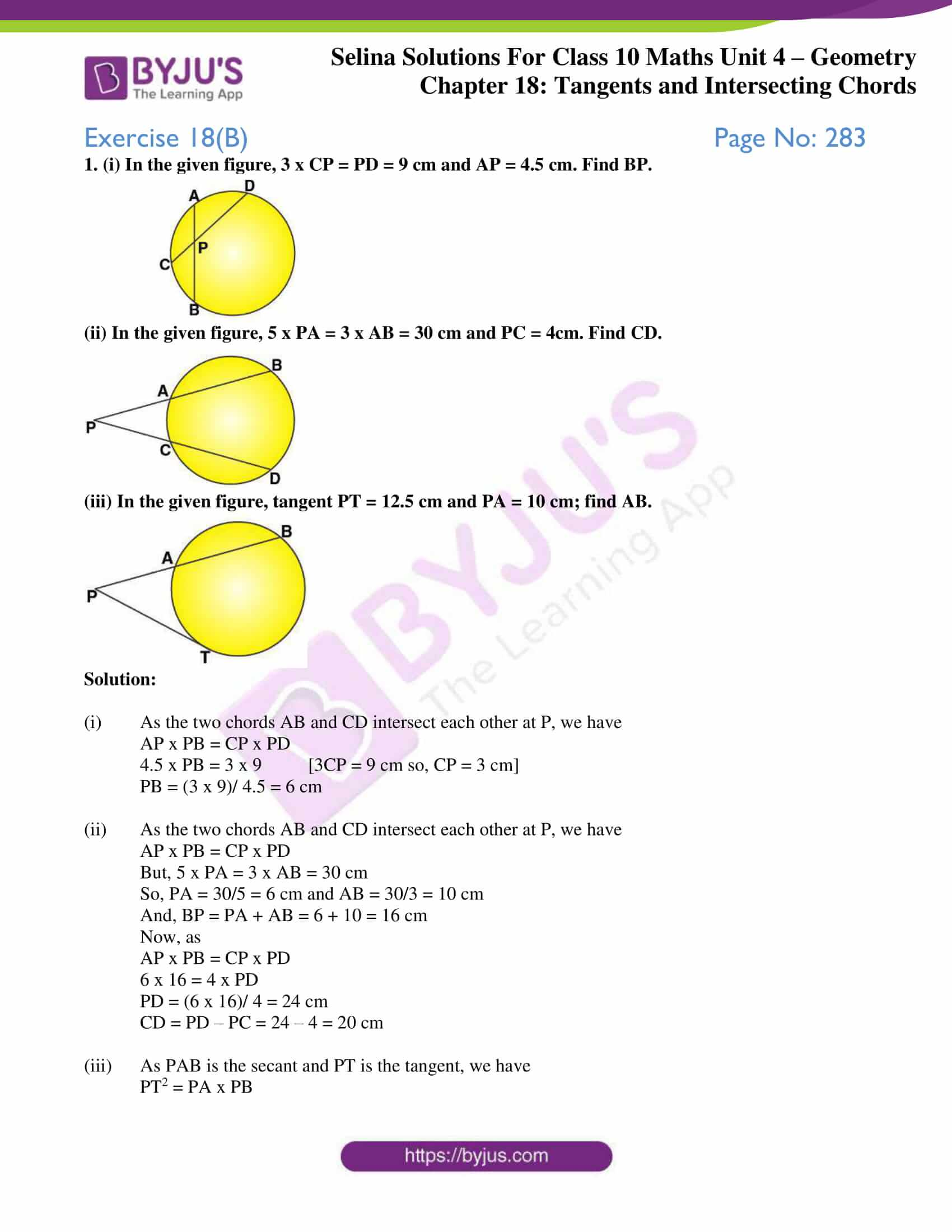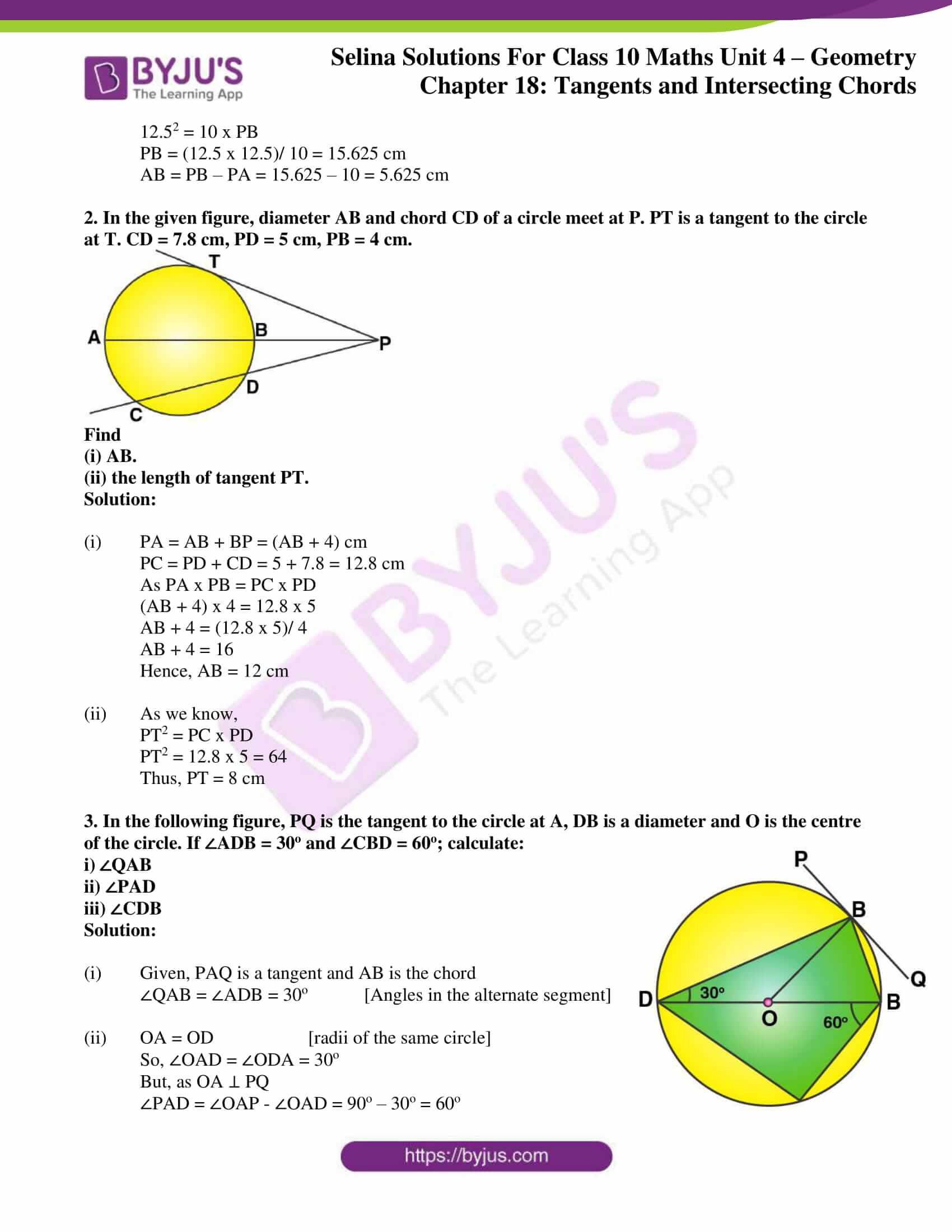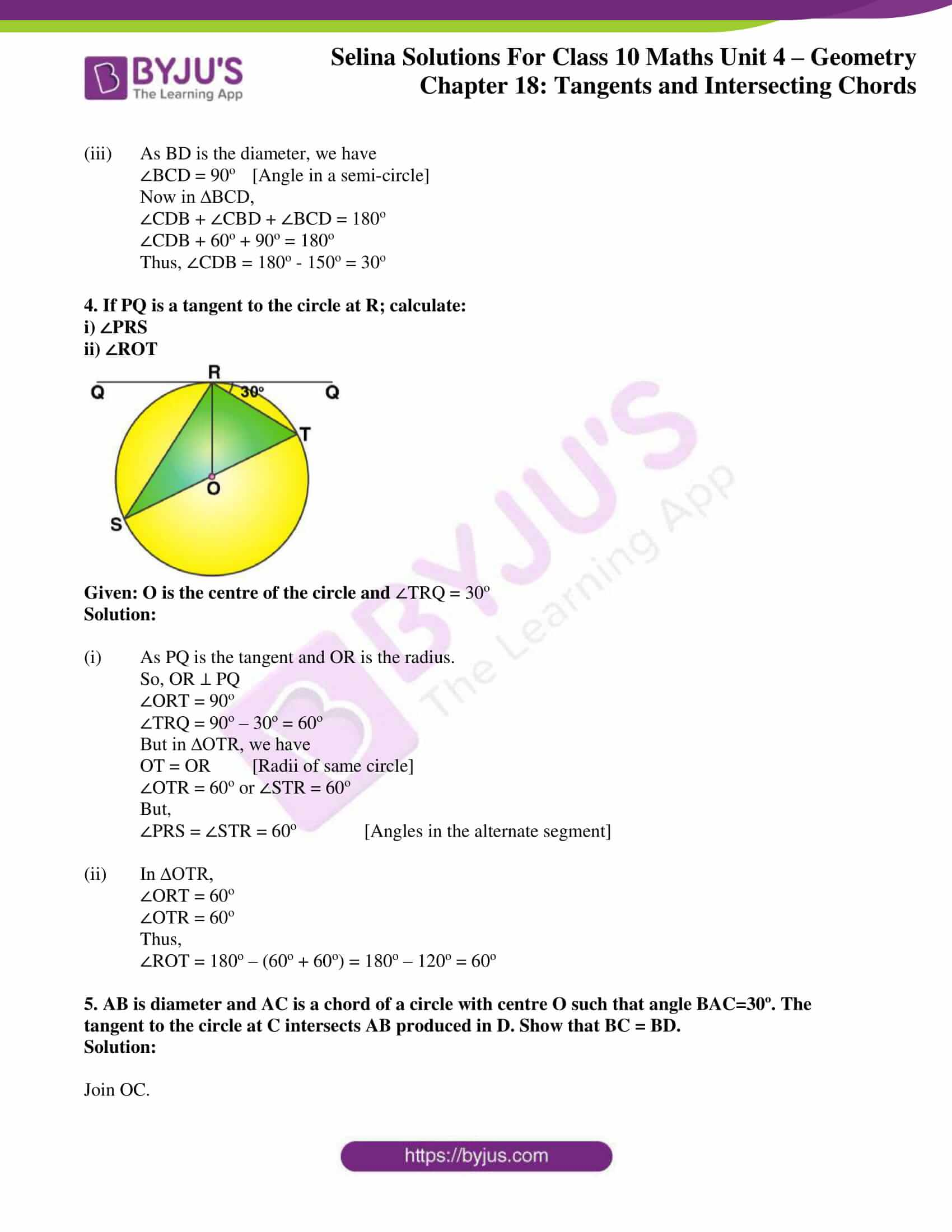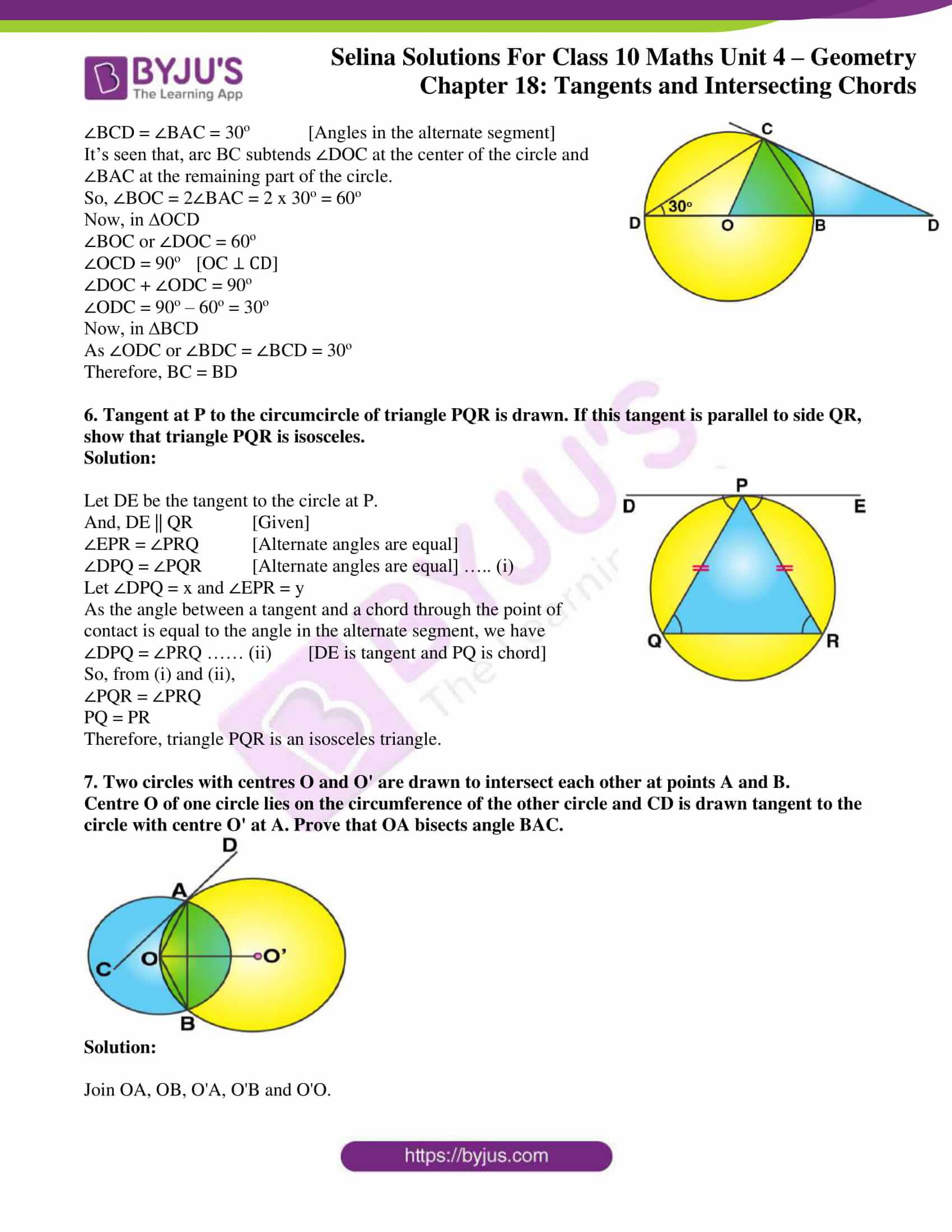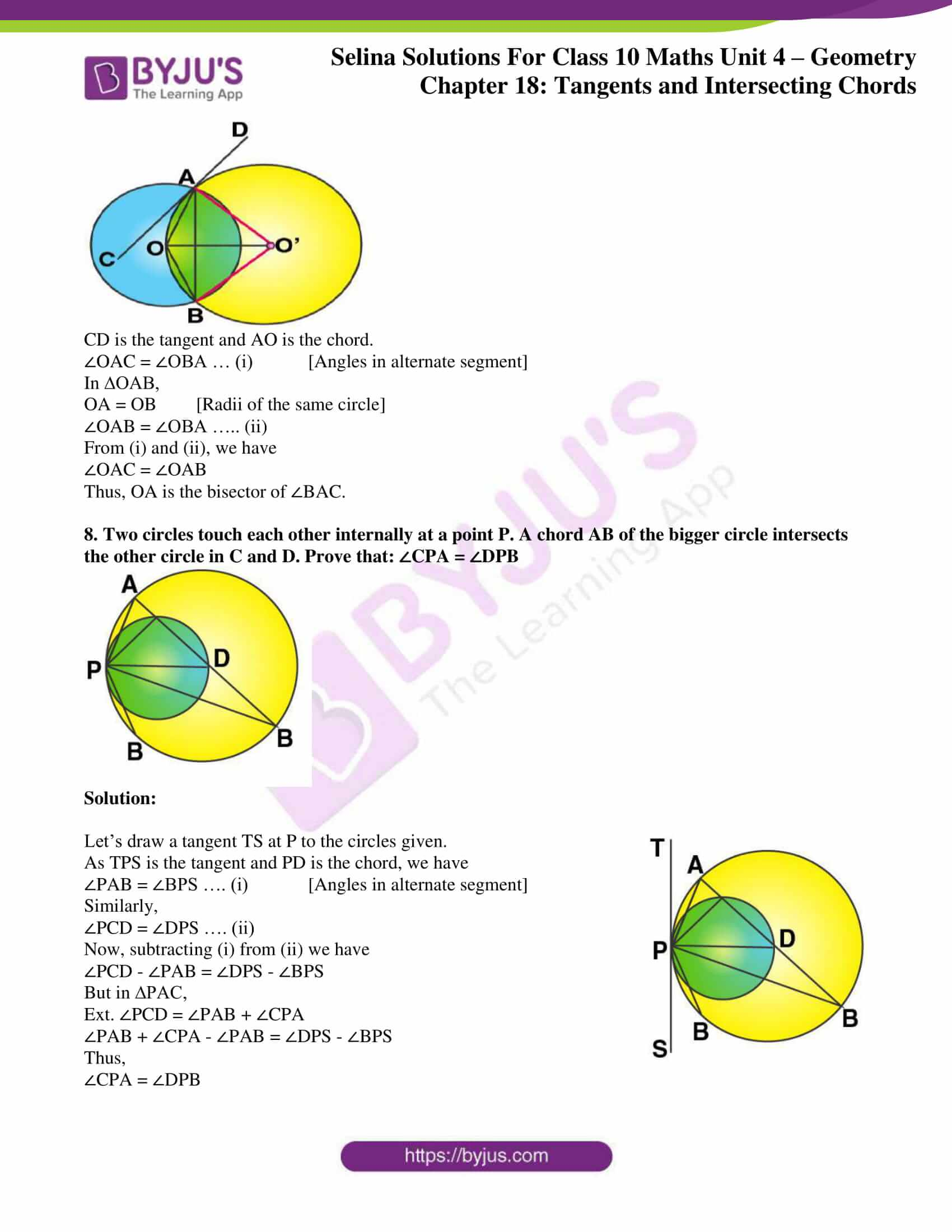### Access Selina Solutions Concise Maths Class 10 Chapter 18 Tangents and Intersecting Chords Exercise 18(B)

1. (i) In the given figure, 3 x CP = PD = 9 cm and AP = 4.5 cm. Find BP.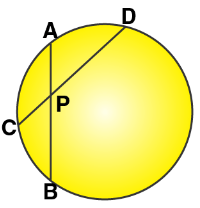(ii) In the given figure, 5 x PA = 3 x AB = 30 cm and PC = 4cm. Find CD.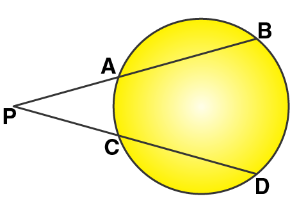(iii) In the given figure, tangent PT = 12.5 cm and PA = 10 cm; find AB.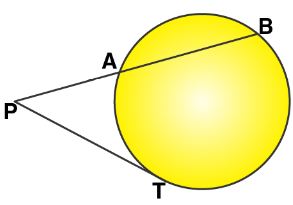Solution:

(i) As the two chords AB and CD intersect each other at P, we have

AP x PB = CP x PD

4.5 x PB = 3 x 9 [3CP = 9 cm so, CP = 3 cm]

PB = (3 x 9)/ 4.5 = 6 cm

(ii) As the two chords AB and CD intersect each other at P, we have

AP x PB = CP x PD

But, 5 x PA = 3 x AB = 30 cm

So, PA = 30/5 = 6 cm and AB = 30/3 = 10 cm

And, BP = PA + AB = 6 + 10 = 16 cm

Now, as

AP x PB = CP x PD

6 x 16 = 4 x PD

PD = (6 x 16)/ 4 = 24 cm

CD = PD – PC = 24 – 4 = 20 cm

(iii) As PAB is the secant and PT is the tangent, we have

PT2 = PA x PB

12.52 = 10 x PB

PB = (12.5 x 12.5)/ 10 = 15.625 cm

AB = PB – PA = 15.625 – 10 = 5.625 cm

2. In the given figure, diameter AB and chord CD of a circle meet at P. PT is a tangent to the circle at T. CD = 7.8 cm, PD = 5 cm, PB = 4 cm.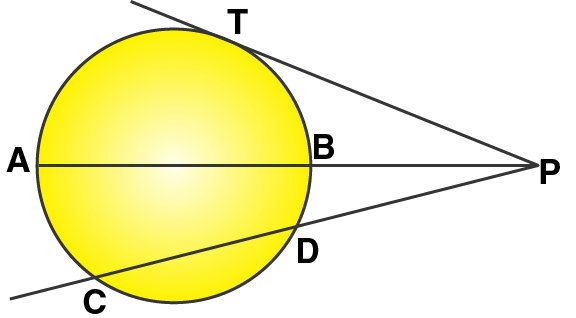Find

(i) AB.

(ii) the length of tangent PT.

Solution:

(i) PA = AB + BP = (AB + 4) cm

PC = PD + CD = 5 + 7.8 = 12.8 cm

As PA x PB = PC x PD

(AB + 4) x 4 = 12.8 x 5

AB + 4 = (12.8 x 5)/ 4

AB + 4 = 16

Hence, AB = 12 cm

(ii) As we know,

PT2 = PC x PD

PT2 = 12.8 x 5 = 64

Thus, PT = 8 cm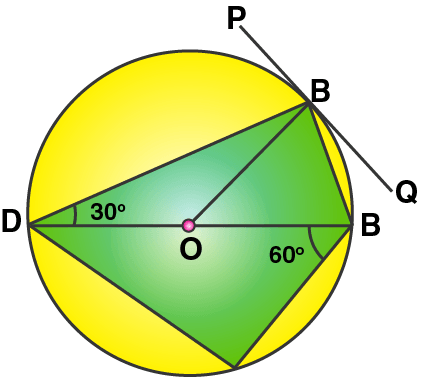3. In the following figure, PQ is the tangent to the circle at A, DB is a diameter and O is the centre of the circle. If ∠ADB = 30o and ∠CBD = 60o; calculate:

i) ∠QAB

iii) ∠CDB

Solution:

(i) Given, PAQ is a tangent and AB is the chord

∠QAB = ∠ADB = 30o [Angles in the alternate segment]

(ii) OA = OD [radii of the same circle]

So, ∠OAD = ∠ODA = 30o

But, as OA ⊥ PQ

(iii) As BD is the diameter, we have

∠BCD = 90o [Angle in a semi-circle]

Now in ∆BCD,

∠CDB + ∠CBD + ∠BCD = 180o

∠CDB + 60o + 90o = 180o

Thus, ∠CDB = 180o – 150o = 30o

4. If PQ is a tangent to the circle at R; calculate:

i) ∠PRS

ii) ∠ROT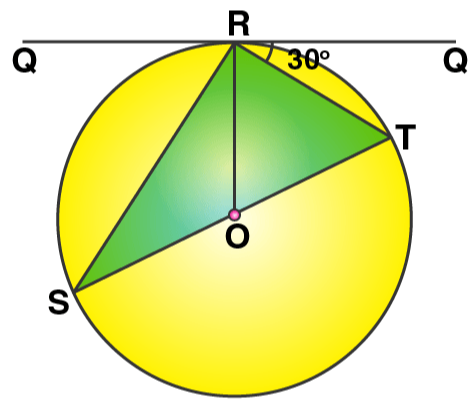Given: O is the centre of the circle and ∠TRQ = 30o

Solution:

(i) As PQ is the tangent and OR is the radius.

So, OR ⊥ PQ

∠ORT = 90o

∠TRQ = 90o – 30o = 60o

But in ∆OTR, we have

OT = OR [Radii of same circle]

∠OTR = 60o or ∠STR = 60o

But,

∠PRS = ∠STR = 60o [Angles in the alternate segment]

(ii) In ∆OTR,

∠ORT = 60o

∠OTR = 60o

Thus,

∠ROT = 180o – (60o + 60o) = 180o – 120o = 60o

5. AB is diameter and AC is a chord of a circle with centre O such that angle BAC=30º. The tangent to the circle at C intersects AB produced in D. Show that BC = BD.

Solution:

Join OC.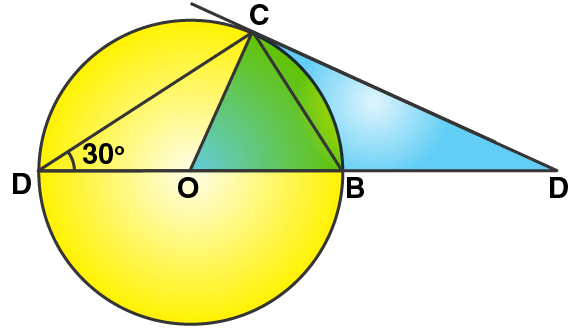∠BCD = ∠BAC = 30o [Angles in the alternate segment]

It’s seen that, arc BC subtends ∠DOC at the center of the circle and ∠BAC at the remaining part of the circle.

So, ∠BOC = 2∠BAC = 2 x 30o = 60o

Now, in ∆OCD

∠BOC or ∠DOC = 60o

∠OCD = 90o [OC ⊥ CD]

∠DOC + ∠ODC = 90o

∠ODC = 90o – 60o = 30o

Now, in ∆BCD

As ∠ODC or ∠BDC = ∠BCD = 30o

Therefore, BC = BD

6. Tangent at P to the circumcircle of triangle PQR is drawn. If this tangent is parallel to side QR, show that triangle PQR is isosceles.

Solution: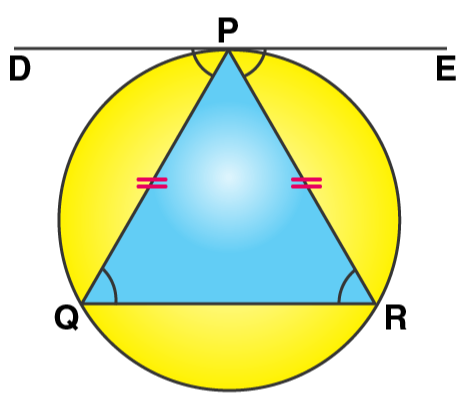Let DE be the tangent to the circle at P.

And, DE || QR [Given]

∠EPR = ∠PRQ [Alternate angles are equal]

∠DPQ = ∠PQR [Alternate angles are equal] ….. (i)

Let ∠DPQ = x and ∠EPR = y

As the angle between a tangent and a chord through the point of contact is equal to the angle in the alternate segment, we have

∠DPQ = ∠PRQ …… (ii) [DE is tangent and PQ is chord]

So, from (i) and (ii),

∠PQR = ∠PRQ

PQ = PR

Therefore, triangle PQR is an isosceles triangle.

7. Two circles with centres O and O’ are drawn to intersect each other at points A and B.

Centre O of one circle lies on the circumference of the other circle and CD is drawn tangent to the circle with centre O’ at A. Prove that OA bisects angle BAC.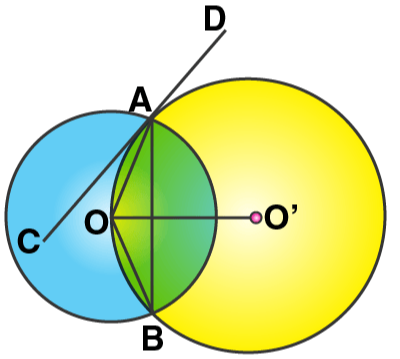Solution:

Join OA, OB, O’A, O’B and O’O.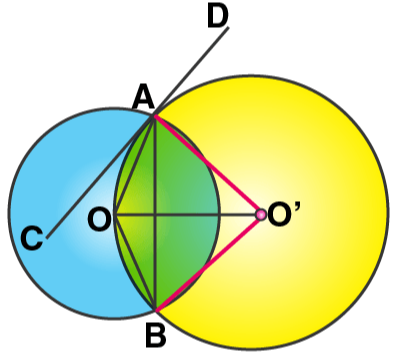CD is the tangent and AO is the chord.

∠OAC = ∠OBA … (i)  [Angles in alternate segment]

In ∆OAB,

OA = OB [Radii of the same circle]

∠OAB = ∠OBA ….. (ii)

From (i) and (ii), we have

∠OAC = ∠OAB

Thus, OA is the bisector of ∠BAC.

8. Two circles touch each other internally at a point P. A chord AB of the bigger circle intersects the other circle in C and D. Prove that: ∠CPA = ∠DPB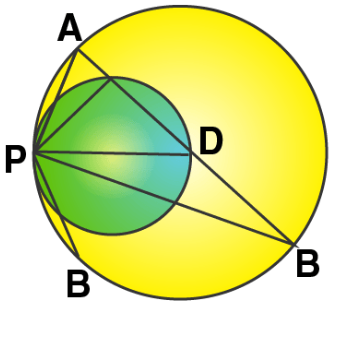Solution: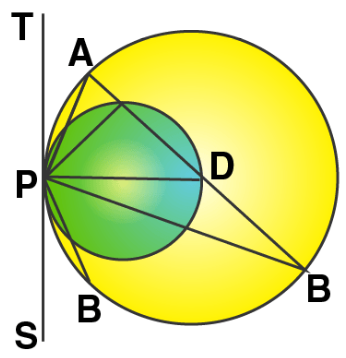Let’s draw a tangent TS at P to the circles given.

As TPS is the tangent and PD is the chord, we have

∠PAB = ∠BPS …. (i) [Angles in alternate segment]

Similarly,

∠PCD = ∠DPS …. (ii)

Now, subtracting (i) from (ii) we have

∠PCD – ∠PAB = ∠DPS – ∠BPS

But in ∆PAC,

Ext. ∠PCD = ∠PAB + ∠CPA

∠PAB + ∠CPA – ∠PAB = ∠DPS – ∠BPS

Thus,

∠CPA = ∠DPB